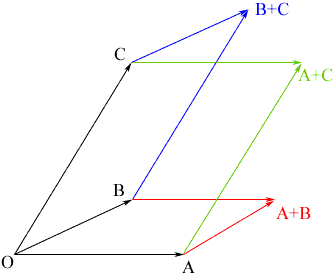Quandaries and Queries Hi, my name is Aman and  I'm a secondary grade twelve student. I came across a question while doing my homework and I need some help. I'm taking a university math course ( Geometry and Discrete math) The question is: " If OA, OB, and OC are three edges of a parallelepiped where O is (0,0,0), A is (2,4,-2), B is (3,6,1), and C is (4,0,-1), find the coordinates of the other vertices of the parallelepiped.)"  ( Crippin, page 173). This question came out of The Harcourt Mathematics 12 text book. Please help me and email me back asap. I have a test in a few days!!!! Thank you, Hi Aman, Look at a cardboard box and imagine that one of the corners is O and the ends of the three edges that start at that corner are A, B and C. This is a rectangular parallelepiped but it is an approximate model of what you have. I don't know the book you mention but I am going to assume that you have learned about vectors. I see the three edges of the parallelepiped OA, OB and OC as vectors from O to the other three points. I then looked at the three vectors A+B, B+C and A+C. There is a diagram below.You can find each of these vectors, in particular A+B = (2,4,-2) + (3,6,1) = (5,10,-1) Now you have 7 of the 8 vertices of the parallelepiped, O, A, B, C, A+B, B+C and A+C. Again I see A, B, C, A+B, B+C and A+C as vectors form O. Can you see how to use two of these vectors to find the 8th vertex? Penny Go to Math Central